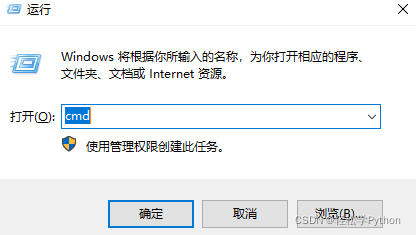﻿ Python实现学生管理系统并生成exe可执行文件详解流程_python_脚本之家
python# Python实现学生管理系统并生成exe可执行文件详解流程## 一、准备工作

• Python 3.8
• Pycharm 2021.2

• Python基础语法
• 基本的数据类型与结构
• 基本的逻辑控制语句
• 实战小项目

## 二、代码流程

= 赋值 就是把等号左边的内容 用 等号右边的这个变量名字 接收

```msg = """**************************************************

1. 新建学生信息
2. 显示全部信息
3. 查询学生信息
4. 删除学生信息
5. 修改学生信息

0. 退出系统
**************************************************"""
```

```student_info = [
{'姓名': '木子', '语文': 60, '数学': 60, '英语': 60, '总分': 180},
{'姓名': '巳月', '语文': 60, '数学': 60, '英语': 60, '总分': 180},
{'姓名': '婧琪', '语文': 60, '数学': 60, '英语': 60, '总分': 180},
]
```

```while True:
print(msg)  # 输出函数 print
# = 左边都是自定义的变量  变量不能以数字开头 不推荐使用关键字作为变量名 建议变量见名知意
# 输入的内容 数据类型是字符串 数据类型
input_world = input('请输入你想要进行的操作: ')
# print('你选择的操作是: ', input_world)
# 多条件判断的语句 一个 = 是赋值  两个 = 比较 是否相等
# 注释
if input_world == '1': # 判断 input_world(输入的内容) 是否 等于 1
print('新建学生信息')
name = input('请输入学生的姓名: ')
chinese = input('请输入学生的语文成绩: ')
math = input('请输入学生的数学成绩: ')
English = input('请输入学生的英语成绩: ')
# len() == 10 统计元素个数 如果 多或者少 进行判断
total = int(chinese) + int(math) + int(English)  # 总分 转整型
dit = {
'姓名': name,
'语文': chinese,
'数学': math,
'英语': English,
'总分': total,
}
student_info.append(dit)

elif input_world == '2':
print('显示全部信息')
print('姓名\t语文\t数学\t英语\t总分')
```

for遍历循环

```for student in student_info:
# 隔空 空tab键位 四个空格 \n换行
# {} 字典数据类型  根据键值对提取内容 (根据冒号左边的内容 提取冒号右边的内容)
# 字典取值的方法  student['语文']
print(student['姓名'] + '\t' +
str(student['语文']) + '\t\t' +
str(student['数学']) + '\t\t' +
str(student['英语']) + '\t\t' +
str(student['总分'])
)
```

```elif input_world == '3':
print('查询学生信息')
input_name = input('请输入你要查询的学生姓名: ')

for student in student_info:
if student['姓名'] == input_name:
print('姓名\t语文\t数学\t英语\t总分')
# 格式整齐
print(student['姓名'] + '\t' +
str(student['语文']) + '\t\t' +
str(student['数学']) + '\t\t' +
str(student['英语']) + '\t\t' +
str(student['总分'])
)
break  # 跳出循环
else:
print('学生信息有误, 查询信息失败')
```

```elif input_world == '4':
print('删除学生信息')
input_name = input('请输入你要删除的学生姓名: ')
for student in student_info:
if student['姓名'] == input_name:
# 列表如何删除元素
# pop 弹出列表元素 并且删除
student_info.remove(student)
print(student['姓名'], '信息删除成功')
break
else:
print('学生信息有误, 删除信息失败')
```

``` elif input_world == '5':
print('修改学生信息')
input_name = input('请输入你要修改的学生姓名: ')
for student in student_info:
if student['姓名'] == input_name:
chinese = input('请重新输入语文成绩: ')
math = input('请重新输入数学成绩: ')
english = input('请重新输入英语成绩: ')
total = int(chinese) + int(math) + int(english)
student['语文'] = chinese
student['数学'] = math
student['英语'] = english
student['总分'] = total
break
else:
print('学生信息有误, 修改信息失败')
```

``` elif input_world == '0':
print('退出系统')
break
```

```msg = """**************************************************

1. 新建学生信息
2. 显示全部信息
3. 查询学生信息
4. 删除学生信息
5. 修改学生信息

0. 退出系统
**************************************************"""

student_info = [
{'姓名': '木子', '语文': 60, '数学': 60, '英语': 60, '总分': 180},
{'姓名': '巳月', '语文': 60, '数学': 60, '英语': 60, '总分': 180},
{'姓名': '婧琪', '语文': 60, '数学': 60, '英语': 60, '总分': 180},
]

# 死循环
while True:
print(msg)  # 输出函数 print
input_world = input('请输入你想要进行的操作: ')
if input_world == '1': # 判断 input_world(输入的内容) 是否 等于 1
print('新建学生信息')
name = input('请输入学生的姓名: ')
chinese = input('请输入学生的语文成绩: ')
math = input('请输入学生的数学成绩: ')
English = input('请输入学生的英语成绩: ')

total = int(chinese) + int(math) + int(English)  # 总分 转整型
dit = {
'姓名': name,
'语文': chinese,
'数学': math,
'英语': English,
'总分': total,
}
student_info.append(dit)

elif input_world == '2':
print('显示全部信息')
print('姓名\t语文\t数学\t英语\t总分')

for student in student_info:

print(student['姓名'] + '\t' +
str(student['语文']) + '\t\t' +
str(student['数学']) + '\t\t' +
str(student['英语']) + '\t\t' +
str(student['总分'])
)

elif input_world == '3':
print('查询学生信息')
input_name = input('请输入你要查询的学生姓名: ')

for student in student_info:
if student['姓名'] == input_name:
print('姓名\t语文\t数学\t英语\t总分')
# 格式整齐
print(student['姓名'] + '\t' +
str(student['语文']) + '\t\t' +
str(student['数学']) + '\t\t' +
str(student['英语']) + '\t\t' +
str(student['总分'])
)
break  # 跳出循环
else:
print('学生信息有误, 查询信息失败')

elif input_world == '4':
print('删除学生信息')
input_name = input('请输入你要删除的学生姓名: ')
for student in student_info:
if student['姓名'] == input_name:
# 列表如何删除元素
# pop 弹出列表元素 并且删除
student_info.remove(student)
print(student['姓名'], '信息删除成功')
break
else:
print('学生信息有误, 删除信息失败')

elif input_world == '5':
print('修改学生信息')
input_name = input('请输入你要修改的学生姓名: ')
for student in student_info:
if student['姓名'] == input_name:
chinese = input('请重新输入语文成绩: ')
math = input('请重新输入数学成绩: ')
english = input('请重新输入英语成绩: ')
total = int(chinese) + int(math) + int(english)
student['语文'] = chinese
student['数学'] = math
student['英语'] = english
student['总分'] = total
break
else:
print('学生信息有误, 修改信息失败')

elif input_world == '0':
print('退出系统')
break

else:
print('请输入正确的操作')
```

## 三、打包exe可执行程序